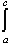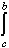#Interactive Real Analysis

Next | Previous | Glossary | Map

## 7.1. Riemann Integral

### Proposition 7.1.12: Properties of the Riemann Integral

Suppose f and g are Riemann integrable functions defined on [a, b]. Then
1.c f(x) + d g(x) dx = cf(x) dx + dg(x) dx
2. If a < c < b thenf(x) dx =f(x) dx +f(x) dx
3. |f(x) dx || f(x) | dx
4. If g is another function defined on [a, b] such that g(x) < f(x) on [a, b], theng(x) dxf(x) dx
5. If g is another Riemann integrable function on [a, b] then f(x) . g(x) is integrable on [a, b]

### Proof:

Next | Previous | Glossary | Map